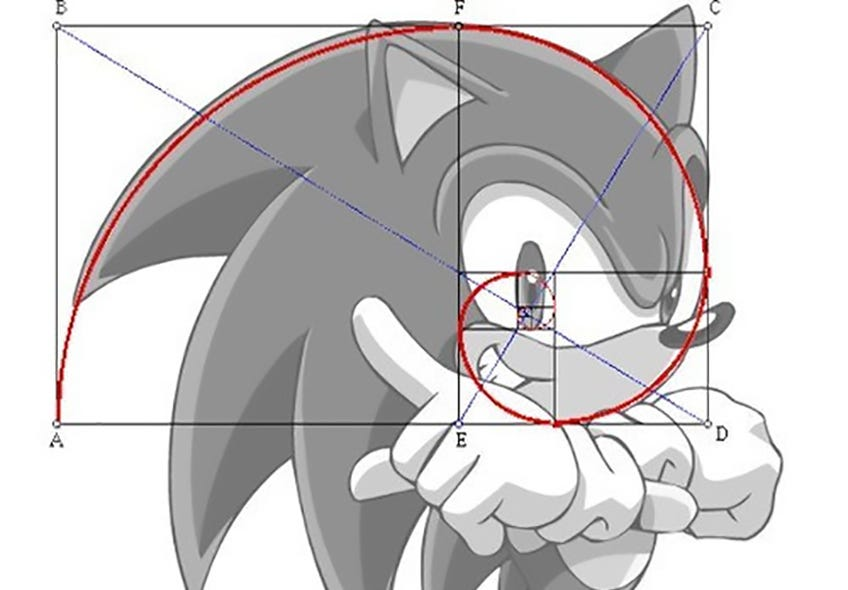# Implementing Memoization in JavascriptThink about how fast you can calculate the shape of Sonic’s face and hair with memoization!

If you’ve ever written an recursive function that computes the same value many times, you may have found yourself wishing that the results of these function calls were stored in some way for fast retrieval. If only it were possible, you might say, for my function to remember the values it has already calculated! Fear not — thanks to the power of closures and hash tables, memoization can help us do just that.

For the CS historians out there, “memoization” (derived from memorandum, Latin for “to be remembered”), was invented by British artificial intelligence pioneer Donald Michie, who first published a scholarly article on the power of “memo” functions in 1968. Essentially, memoization is a technique that stores (“caches”) the results of previous function calls in a data structure like a hash table, so that they can be quickly retrieved as they are called again. The power of this “cache” truly manifests in recursive functions that make many function calls.

For example, take a recursive algorithm to derive the nth number in the fibonacci sequence*. We will want to calculate the values of Fib(n-1) and Fib(n-2) in order to calculate the value of n. Of course, if n is 1or 0, we will simply return n, based on the “seed values” of fibonacci (f(0) = 0, f(1) = 1).

This function is great because of its clarity and brevity — two lines! But think about the work needed to calculate, say, recursiveFib(5):

Already, we are doing repetitive function calls: we calculate recursiveFib(2) three times! This is where memoization comes in handy. Here is the same function using memoization:

Okay, let’s break down what’s going on here:

1. The outer function, memoFib, contains a “memo” object, which will serve as our cache.
2. We then return a function, which has a closure over the “memo” object, and can thus can log the results of its function calls into the “memo.”
3. If the “memo” object contains a key for the value (n) for which we’re calculating fibonacci, we simply return memo[n]
4. If the “memo” object does not contain a key for n, we calculate the fibonacci number for n and save it in the “memo” object as a key-value pair.
5. In both cases, we return memo[n], which is the nth number of the fibonacci sequence.

Using memoization, we can reduce the number of times we run the recursive fibonacci function. In the fibonacci(5) example, the second and third time we call fib(2), we return the cached value from our memo for memo, rather than recalculating it!

Let’s look at another example using another common function: a function to calculate factorial of n:

Once again, an awesome function on surface, but expensive in the long run. Let’s say we’ve calculated recursiveFac(5) and now we want to calculate recursiveFac(6). Without a cache to store our previously calculated values, we have to repeat the entire process, returning the function another 5 times. With memoization, the same function looks like this:

Now, if we call factorial(6) after we’ve called factorial(5), we’ve already saved our calculated value for factorial(5) in our “memo” object, which has been moved outside of the function in order to retain its caching calculations. Thus, we only need to call the function once, calculate 6 * factorial(5), which sends us straight to our “memo” to retrieve the cached value. Awesome!

But wait — if memoization follows the same general format (retrieving values from a cache in the outer scope of the function, or calculating the value using the anonymous closure function), couldn’t we write a general memoize function? Yes we can!

In this function, we’re creating a cache in the outer function that stores the results of the callback function if they do not already exist. We take advantage of closures by using the “arguments” object provided from the anonymous closure function (“callback”), which will not include any arguments in the outer function (“memoize”). We can thus use this generic function to apply memoization to any function, like so:

Some notes:

• You may have noticed that fibonacci and factorial are a “pure” functions — that is, the same input value will always return the same output. This is a necessary condition for using memoization, as our cache needs to remember consistent return values for the inputs.
• Memoization trades time for space — we must create a space in our cache for every input needed to calculate our result.

*The fibonacci sequence is a mathematical sequence of integers where each integer after the first two integers in the sequence are the sum of the two integers before it. For our purposes, we will assume that the two “seed values” (the first two integers of the sequence) are Fib(0) = 0 and Fib(1) = 1. To illustrate, the first 5 numbers in the Fibonacci sequence as defined above are 0, 1, 1, 2, and 3.

--

--